In force

Version from: 01/01/2023
Amendments (1)
Search within this legal act

### Article 261 - Calculation of risk-weighted exposure amounts under the Standardised Approach (SEC-SA)

Article 261

Calculation of risk-weighted exposure amounts under the Standardised Approach (SEC-SA)

1.

Under the SEC-SA, the risk-weighted exposure amount for a position in a securitisation shall be calculated by multiplying the exposure value of the position as calculated in accordance with Article 248 by the applicable risk weight determined as follows, in all cases subject to a floor of 15 %:

 RW = 1 250  % when D ≤ KA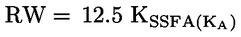when A ≥ KA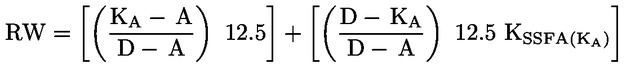when A < KA < D

where:

 D is the detachment point as determined in accordance with Article 256;
 A is the attachment point as determined in accordance with Article 256;
 KA is a parameter calculated in accordance with paragraph 2;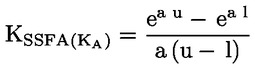where:

 a = – (1/(p · KA))
 u = D – KA
 l = max (A – KA; 0)
 p = 1 for a securitisation exposure that is not a re-securitisation exposure
2.

For the purposes of paragraph 1, KA shall be calculated as follows: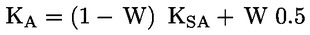where:

KSA is the capital charge of the underlying pool as defined in Article 255;

W = ratio of:

(a)

the sum of the nominal amount of underlying exposures in default, to

(b)

the sum of the nominal amount of all underlying exposures.

For these purposes, an exposure in default shall mean an underlying exposure which is either: (i) 90 days or more past due; (ii) subject to bankruptcy or insolvency proceedings; (iii) subject to foreclosure or similar proceeding; or (iv) in default in accordance with the securitisation documentation.

Where an institution does not know the delinquency status for 5 % or less of underlying exposures in the pool, the institution may use the SEC-SA subject to the following adjustment in the calculation KA: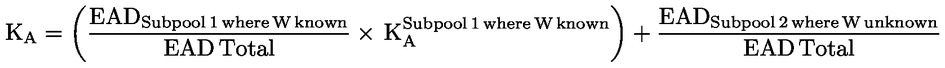Where the institution does not know the delinquency status for more than 5 % of underlying exposures in the pool, the position in the securitisation must be risk-weighted at 1 250  %.

3.
Where an institution has a securitisation position in the form of a derivative to hedge market risks, including interest rate or currency risks, the institution may attribute to that derivative an inferred risk weight equivalent to the risk weight of the reference position calculated in accordance with this Article.

For the purposes of this paragraph, the reference position shall be the position that is pari passu in all respects to the derivative or, in the absence of such pari passu position, the position that is immediately subordinate to the derivative.